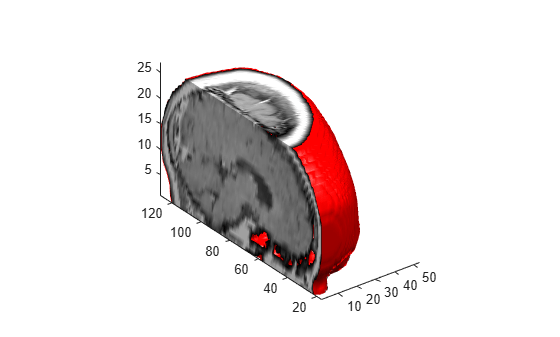# isocaps

Compute isosurface end-cap geometry

## Syntax

```fvc = isocaps(X,Y,Z,V,isovalue) fvc = isocaps(V,isovalue) fvc = isocaps(...,'enclose') fvc = isocaps(...,'whichplane') [f,v,c] = isocaps(...) isocaps(...) ```

## Description

`fvc = isocaps(X,Y,Z,V,isovalue)` computes isosurface end-cap geometry for the volume data `V` at isosurface value `isovalue`. The arrays `X`, `Y`, and `Z` define the coordinates for the volume `V`.

The struct `fvc` contains the face, vertex, and color data for the end-caps and can be passed directly to the `patch` command.

`fvc = isocaps(V,isovalue)` assumes the arrays `X`, `Y`, and `Z` are defined as `[X,Y,Z] = meshgrid(1:n,1:m,1:p)` where ```[m,n,p] = size(V)```.

`fvc = isocaps(...,'enclose') ` specifies whether the end-caps enclose data values above or below the value specified in `isovalue`. The `'enclose'` option can be either `'above'` (default) or `'below'`.

`fvc = isocaps(...,'whichplane')` specifies on which planes to draw the end-caps. Possible values for `'whichplane'` are `'all'` (default), `'xmin'`, `'xmax'`, `'ymin'`, `'ymax'`, `'zmin'`, or `'zmax'`.

`[f,v,c] = isocaps(...)` returns the face, vertex, and color data for the end-caps in three arrays instead of the struct `fvc`.

`isocaps(...)` without output arguments draws a patch with the computed faces, vertices, and colors.

## Examples

collapse all

This example uses a data set that is a collection of MRI slices of a human skull. It illustrates the use of isocaps to draw the end-caps on this cutaway volume.

The red isosurface shows the outline of the volume (skull) and the end-caps show what is inside of the volume.

The patch created from the end-cap data (`p2`) uses interpolated face coloring, which means the gray colormap and the light sources determine how it is colored. The isosurface patch (`p1`) used a flat red face color, which is affected by the lights, but does not use the colormap.

```load mri D = squeeze(D); D(:,1:60,:) = []; p1 = patch(isosurface(D, 5),'FaceColor','red',... 'EdgeColor','none'); p2 = patch(isocaps(D, 5),'FaceColor','interp',... 'EdgeColor','none'); view(3) axis tight daspect([1,1,.4]) colormap(gray(100)) camlight left camlight lighting gouraud isonormals(D,p1)```